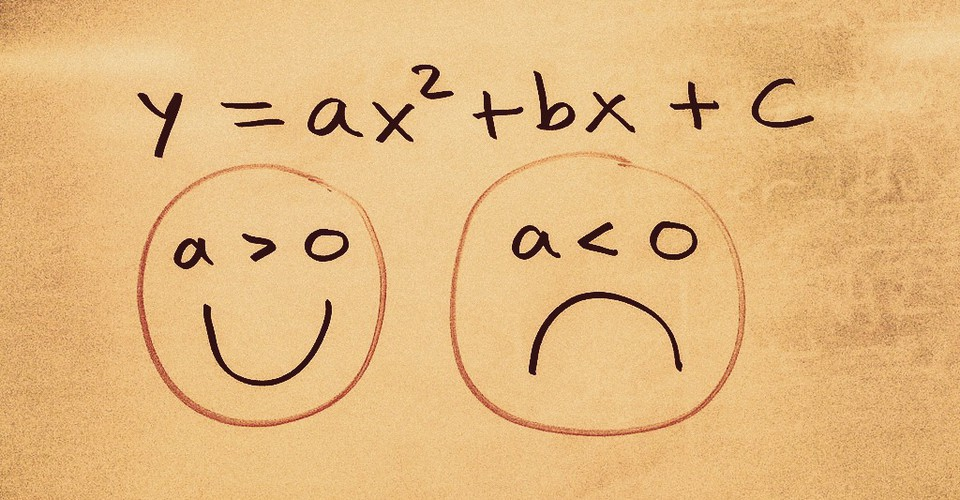# Solving Mathematical Optimization Problems with Differential CalculusLooking at a fundamental use of calculus, and the incredible insight it can provide. Calculus is arguably the most influential mathematical discovery in history, finding uses in physics, economics, engineering, biology, and so much more. Calculus has two branches: Differential calculus; Integral calculus. The former grapples with the rate of change at an instant; the latter with the area between a function and the x-axis. In this article, we will be looking at the differential flavour and, more importantly, at one of its many applications: optimization. Optimization involves finding either the minimum or maximum of a function. You can use this technique whenever you…...